#Function Repository Resource:

# BitStringRowReduce

A memory efficient form of Gaussian elimination to row echelon form modulo 2

Contributed by: Daniel Lichtblau
 ResourceFunction["BitStringRowReduce"][{n1, n2, …},ncols] treats the integers ni as vectors of zeros and ones, each having ncols bits, and does row reduction using mod 2 arithmetic.

## Details and Options

The resulting integers represent bit strings that correspond to the reduced echelon form one would obtain using RowReduce with the option setting Modulus2 on the bit vectors that correspond to the input.
The number of columns must be made explicit since there might be leading zeros in all rows.
The first nonzero bit in each result integer corresponds to a pivot position so the integers in the result will be strictly decreasing in value.
ResourceFunction["BitStringRowReduce"] is intended for dense linear algebra. Sparse methods might be better suited for linear algebra modulo 2 when most matrix entries are zeros.

## Examples

### Basic Examples

Reduce a list of integers bit-wise:

 In:=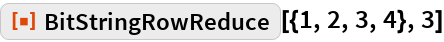Out=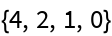Create a random matrix of bit vectors:

 In:=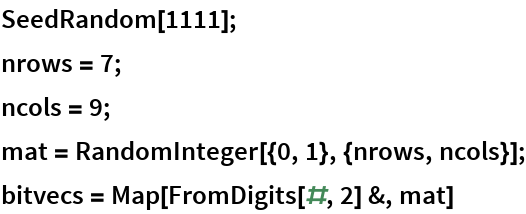Out=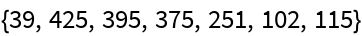Now reduce it:

 In:=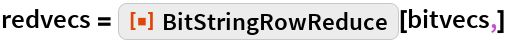Out=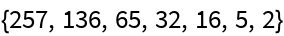### Scope

Create a large array of long integers:

 In:=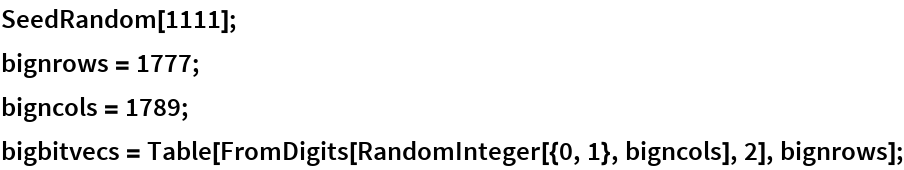ResourceFunction["BitStringRowReduce"] is especially useful when working with matrices that might otherwise require excessive memory:

 In:=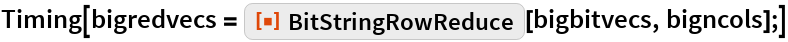Out=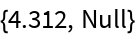### Properties and Relations

Here is the matrix from which the bit vectors were created:

 In:=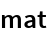Out=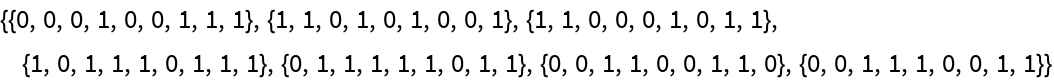Use RowReduce modulo 2 to put it into echelon form:

 In:=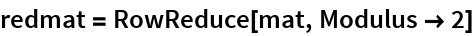Out=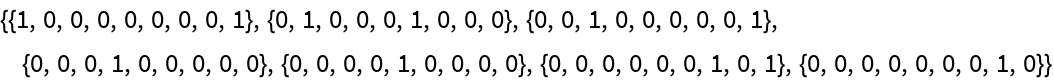Check that this agrees with the result from ResourceFunction["BitStringRowReduce"]:

 In:=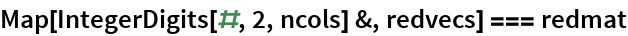Out=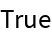Create a matrix of 0‐1 vectors corresponding to the larger integers:

 In:=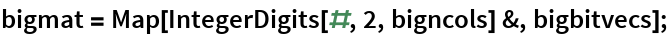RowReduce is substantially slower as compared to ResourceFunction["BitStringRowReduce"]:

 In:=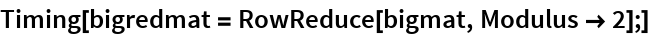Out=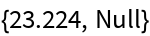Again check that the results are equivalent:

 In:=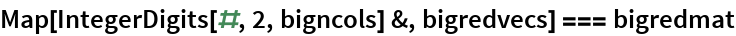Out=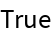Here are the sizes in bytes of the input and result using the bit string approach:

 In:=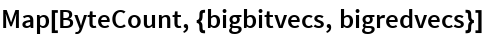Out=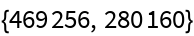Here are the corresponding sizes in bytes of the input and result when using an explicit matrix:

 In:=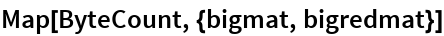Out=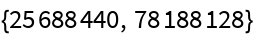## Requirements

Wolfram Language 11.3 (March 2018) or above